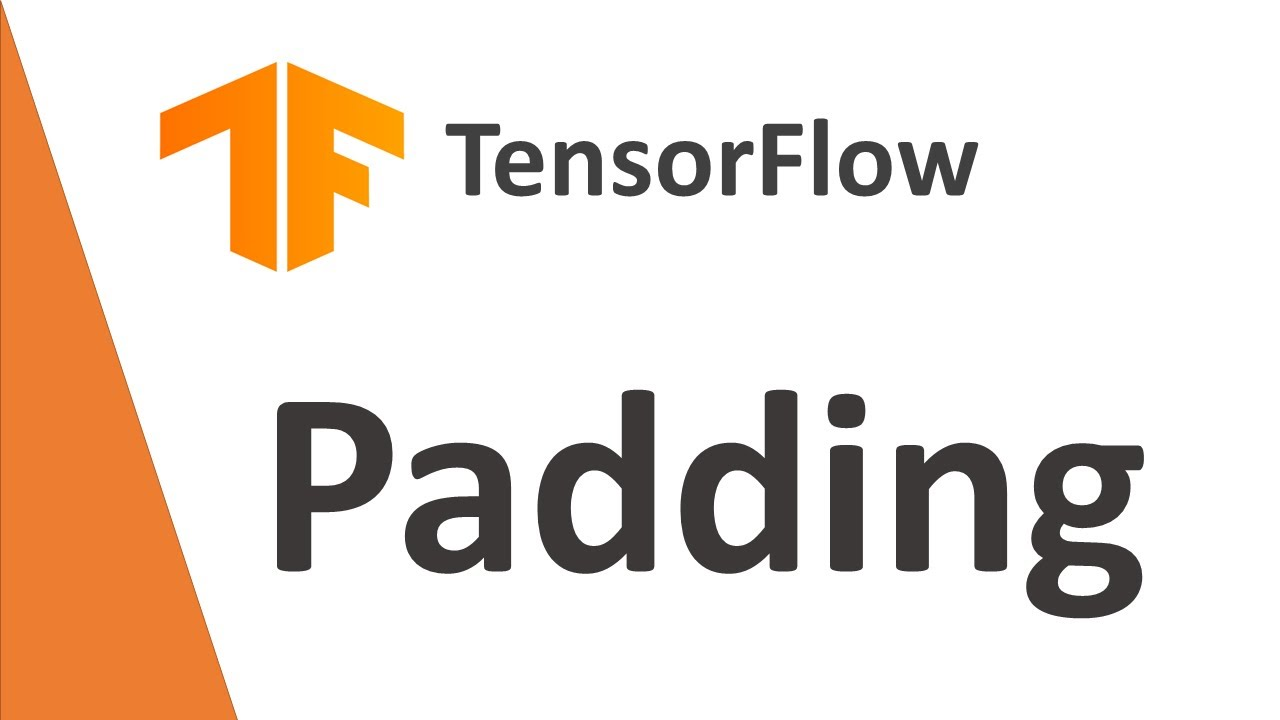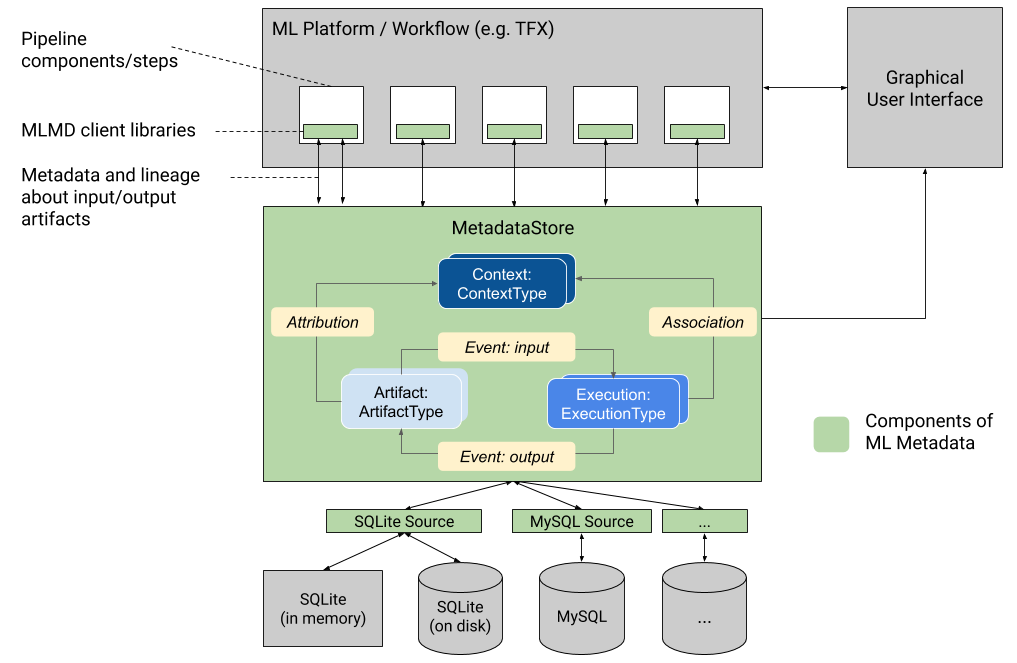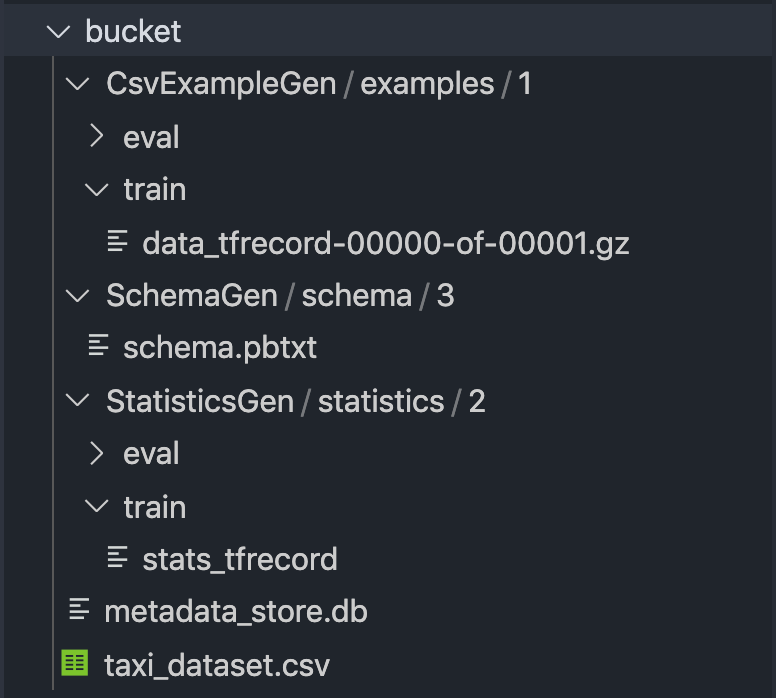1622219692

#tensorflow

## Buddha Community1623139838

## Transfer Learning on Images with Tensorflow 2 – Predictive Hacks

In this tutorial, we will provide you an example of how you can build a powerful neural network model to classify images of **cats **and dogs using transfer learning by considering as base model a pre-trained model trained on ImageNet and then we will train additional new layers for our cats and dogs classification model.

### The Data

We will work with a sample of 600 images from the Dogs vs Cats dataset, which was used for a 2013 Kaggle competition.

#python #transfer learning #tensorflow #images #transfer learning on images with tensorflow #tensorflow 21595417760

## Why it exists and how it’s used in Beam Pipeline ComponentsML Metadata (MLMD) is a library for recording and retrieving metadata associated with ML developer and data scientist workflows.

TensorFlow Extended (TFX) is an end-to-end platform for deploying production ML pipelines

The current version of ML Metadata by the time this article is being published is **v0.22 **(tfx is also v0.22). The API is mature enough to allow for mainstream usage and deployment on the public cloud. Tensorflow Extended uses this extensively for component — component communication, lineage tracking, and other tasks.

We are going to run a very simple pipeline that is just going to generate statistics and the schema for a sample csv of the famous Chicago Taxi Trips dataset. It’s a small ~10mb file and the pipeline can run locally.

``````PIPELINE_ROOT = '<your project root>/bucket' # pretend this is a storage bucket in the cloud
STAGING = 'staging'
TEMP = 'temp'

PROJECT_ID = ''
JOB_NAME = ''

DATASET_PATTERN = 'taxi_dataset.csv'

BEAM_ARGS = [
'--runner=DirectRunner'
]

def create_pipeline():
no_eval_config = example_gen_pb2.Input(splits=[
example_gen_pb2.Input.Split(name='train', pattern=DATASET_PATTERN),
])
example_gen = CsvExampleGen(input=external_input(
PIPELINE_ROOT), input_config=no_eval_config)
statistics_gen = StatisticsGen(examples=example_gen.outputs['examples'])
schema_gen = SchemaGen(statistics=statistics_gen.outputs['statistics'])

return pipeline.Pipeline(
pipeline_name=f'Pipeline {JOB_NAME}',
pipeline_root=PIPELINE_ROOT,
components=[example_gen, statistics_gen, schema_gen],
beam_pipeline_args=BEAM_ARGS,
)

if __name__ == '__main__':
BeamDagRunner().run(create_pipeline())
view raw
metadata_local.py hosted with ❤ by GitHub
``````Generated Artifact List

Run it once and open up the `metadata_store.db` file for inspection.

#metadata #deep-learning #tensorflow #tensorflow-extended #machine-learning #deep learning1598891580

## Google Reveals "What is being Transferred” in Transfer Learning

Recently, researchers from Google proposed the solution of a very fundamental question in the machine learning community — What is being transferred in Transfer Learning? They explained various tools and analyses to address the fundamental question.

The ability to transfer the domain knowledge of one machine in which it is trained on to another where the data is usually scarce is one of the desired capabilities for machines. Researchers around the globe have been using transfer learning in various deep learning applications, including object detection, image classification, medical imaging tasks, among others.

#developers corner #learn transfer learning #machine learning #transfer learning #transfer learning methods #transfer learning resources1597385100

## Overview

There exist two popular integrations of quantum computing packages in standard deep learning libraries:

1. Tensorflow and Cirq as Tensorflow Quantum
2. Pytorch and Qiskit

## Introduction

Quantum machine learning has an interesting application of assisting classical neural networks with quantum layers that involve computation not realisable classically. Recent work in academia has stressed on applications of _quantum-assisted deep learning _which can have complex activations, better representation, and other salient features not achievable in classical networks.

For the implementation side, this means finding a way to integrate quantum processing in normal deep neural networks. Several ways exist to achieve this. Here, we discuss integrating Qiskit as subclasses of Keras layers. Let’s get started.

## Defining the quantum layer

This obviously depends on specific application. The thing to keep in mind is to be consistent in the inputs and outputs this layer has. With `eager_execution` as default in Tensorflow 2.x, it is only natural to fall back to `numpy` arrays as our default inputs to and outputs from all quantum layers.

``````from qiskit.aqua.operators import Z, Y, X
from qiskit.aqua.operators import StateFn

QUBITS = 4
operatorZ = Z ^ Z ^ Z ^ Z
operatorX = X ^ X ^ X ^ X
operatorY = Y ^ Y ^ Y ^ Y

def quantum_layer(initial_parameters):
## expecting parameters to be a numpy array
quantumRegister = QuantumRegister(QUBITS)
quantumCircuit = QuantumCircuit(quantumRegister)

quantumCircuit.h(range(4))

for i in range(len(initial_parameters)):
quantumCircuit.ry(initial_parameters[i] * np.pi, i)

psi = StateFn(quantumCircuit)

## two ways of doing the same thing
expectationX = (~psi @ operatorX @ psi).eval()
expectationY = (~psi @ operatorY @ psi).eval()

expectationZ = np.abs(np.real(expectationZ))
expectations = [expectationX, expectationY, expectationZ,
expectationX + expectationY + expectationZ]

return np.array(expectations)
view raw
quantum_layer.py hosted with ❤ by GitHub
``````

Sample quantum layer

This is an arbitrary quantum layer taking in four inputs and outputting a numpy array of length 4. We calculate the expectations of standard Pauli operators, create a list, and return it. This layer would change according to the specifics of the underlying application.

#tensorflow #deep-learning #quantum-computing #qiskit #machine-learning #deep learning1591262400